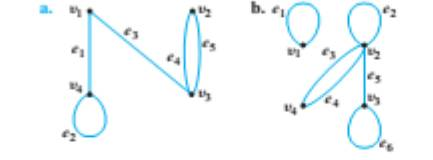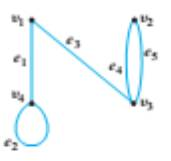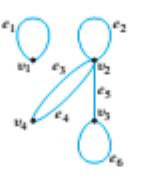Chapter 10.2, Problem 4ES### Discrete Mathematics With Applicat...

5th Edition
EPP + 1 other
ISBN: 9781337694193

#### Solutions

Chapter
Section### Discrete Mathematics With Applicat...

5th Edition
EPP + 1 other
ISBN: 9781337694193
Textbook Problem
6 views

# Find adjacency matrices for the following (undirected) graphs.c. K4, the complete graph on four vertices d. K2,3, the complete bipartite graph on (2, 3) vertices

To determine

(a)

Find adjacency matrices for the (undirected) graph:Explanation

Given information:

Calculation:

The adjacency matrix A = [aij] is n×n zero-one matrix with

aij={1                     if there is an edge from  v i to  v j0                    otherwise

A complete graph Kn(n1) is a simple graph with n vertices and an edge between every pair of vertices.

A complete bipartite graph Km,n with a set M of m vertices and another set N with n vertices, while there are edges between every vertex in M and every vertex of N.

The given graph contains 4 vertices: v1,v2,v3,v4. The adjacency matrix corresponding to the graph then needs to be a 4×4-matrix.

v 1 v 2 v 3 v 4 v 1 v 2 v 3 v 4 [ .... .... .... .... .... .... .... .... .... .... .... .... .... .... .... .... ]

Since e1 is an edge between v1 and v4, we place a 1 in the cell a14 and weplace a 1 in the cell a41

To determine

(b)

Find adjacency matrices for the following (undirected) graph:To determine

(c)

Find adjacency matrices for  K4, the complete graph on four vertices.

To determine

(d)

Find adjacency matrices for K2,3, the complete bipartite graph on (2,3) vertices.

### Still sussing out bartleby?

Check out a sample textbook solution.

See a sample solution

#### The Solution to Your Study Problems

Bartleby provides explanations to thousands of textbook problems written by our experts, many with advanced degrees!

Get Started